Balbharti Maharashtra State Board 12th Chemistry Textbook Solutions Chapter 4 Chemical Thermodynamics Textbook Exercise Questions and Answers.

## Maharashtra State Board Class 12 Chemistry Solutions Chapter 4 Chemical Thermodynamics

1. Select the most appropriate option.

Question 1.
The correct thermodynamic conditions for the spontaneous reaction at all temperatures are
(a) ΔH < 0 and ΔS > 0
(b) ΔH > 0 and ΔS < 0
(c) ΔH < 0 and ΔS < 0
(d) ΔH < 0 and ΔS = 0
(a) ΔH < 0 and ΔS > 0

Question ii.
A gas is allowed to expand in a well-insulated container against a constant external pressure of 2.5 bar from an initial volume of 2.5 L to a final volume of 4.5 L. The change in internal energy, ΔU of the gas will be
(a) -500 J
(b) +500J
(c) -1013 J
(d) +1013 J
(a) -500 J

Question iii.
In which of the following, entropy of the system decreases ?
(a) Crystallisation of liquid into solid
(b) Temperature of crystalline solid is increased from 0 K to 115 K
(c) H2(g) → 2H(g)
(d) 2NaHCO3(s) → Na2CO3(s) + CO2(g) + H2O(g)
(a) Crystallisation of liquid into solid

Question iv.
The enthalpy of formation for all elements in their standard states is
(a) unity
(b) zero
(c) less than zero
(d) different elements
(b) zeroQuestion v.
Which of the following reactions is exothermic ?
(a) H2(g) → 2H(g)
(b) C(s) → C(g)
(c) 2Cl(g) → Cl2(g)
(d) H2O(s) → H2O(l)
(c) 2Cl(g) → Cl2(g)

Question vi.
6.24 g of ethanol are vaporized by supplying 5.89 kJ of heat. Enthalpy of vaporization of ethanol will be
(a) 43.4 kJ mol-1
(b) 60.2 kJ mol-1
(c) 38.9 kJ mol-1
(d) 20.4 kJ mol-1
(a) 43.4 kJ mol-1

Question vii.
If the standard enthalpy of formation of methanol is -238.9 kJ mol-1 then entropy change of the surroundings will be
(a) -801.7 JK-1
(b) 801.7 JK-1
(c) 0.8017 JK-1
(d) -0.8017 JK-1
(b) 801.7 JK-1

Question viii.
Which of the following are not state functions ?
1. Q + W 2. Q 3. W 4. H-TS
(a) 1, 2 and 3
(b) 2 and 3
(c) 1 and 4
(d) 2, 3 and 4
(b) 2 and 3

Question ix.
For vaporization of water at 1 bar, ΔH = 40.63 kJ mol-1 and ΔS =108.8 JK-1 mol-1. At what temperature, ΔG = 0?
(a) 273.4 K
(b) 393.4 K
(c) 373.4 K
(d) 293.4 K
(c) 373.4 K

Question x.
Bond enthalpies of H – H, Cl – Cl and H – Cl bonds are 434 kJ mol-1, 242 kJ mol-2 and 431 kJ mol-1, respectively. Enthalpy of formation of HCl is
(a) 245 kJ mol-1
(b) -93 kJ mol-1
(c) -245 kJ mol-1
(d) 93 kJ mol-1
(b) -93 kJ mol-12. Answer the following in one or two sentences.

Question i.
Comment on the statement: No work is involved in an expansion of a gas in vacuum.
(1) When a gas expands against an external pressure Pex, changing the volume from V1 to V2, the work obtained is given by
W = -Pex (V2 – V1).
(2) Hence the work is performed by the system when it experiences the opposing force or pressure.
(3) Greater the opposing force, more is the work.
(4) In free expansion, the gas expands in vaccum where it does not experience opposing force, (P = 0). Since external pressure is zero, no work is obtained.
∴ W = -Pex (V2 – V1)
= -0 × (V2 – V1)
= 0
(5) Since during expansion in vacuum no energy is expended, it is called free expansion.

Question ii.
State the first law of thermodynamics.
The first law of thermodynamics is based on the principle of conservation of energy and can be stated in different ways as follows :

1. Energy can neither be created nor destroyed, however, it may be converted from one form into another.
2. Whenever, a quantity of one kind of energy is consumed or disappears, an equivalent amount of another kind of energy appears.
3. The total mass and energy of an isolated system remain constant, although there may be interconservation of energy from one form to another.
4. The total energy of the universe remains constant.

Question iii.
What is enthalpy of fusion?
Enthalpy of fusion (ΔfusH) : The enthalpy change that accompanies the fusion of one mole of a solid into a liquid at constant temperature and pressure is called enthalpy of fusion.
For example,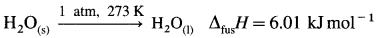This equation describes that when one mole of ice melts (fuses) at 0 °C (273 K) and 1 atmosphere, 6.1 kJ of heat will be absorbed.

Question iv.
What is standard state of a substance?
The thermodynamic standard state of a substance (compound) is the most stable physical state of it at 298 K and 1 atmosphere (or 1 bar). The enthalpy of the substance in the standard state is represented as ΔfH0.Question v.
State whether ∆S is positive, negative or zero for the reaction 2H(g) → H2(g). Explain.
(i) The given reaction, 2H(g) → H2(g) is the formation of H2(g) from free atoms.
(ii) Since two H atoms form one H2 molecule, ∆n = 1 – 2= -1 and disorder is decreased. Hence entropy change ∆S < 0 (or negative).

Question vi.
State second law of thermodynamics in terms of entropy.
The second law of thermodynamics states that the total entropy of the system and its surroundings (universe) increases in a spontaneous process.
OR
Since all the natural processes are spontaneous, the entropy of the universe increases.
It is expressed mathematically as
∆ STotal = ∆ Ssystem + ∆Ssurr > 0
∆ SUniverse = ∆ Ssystem + ∆ Ssurr > 0

Question vii.
If the enthalpy change of a reaction is ∆H how will you calculate entropy of surroundings?
(i) For endothermic reaction, ∆H > 0. This shows the system absorbs heat from surroundings.
∴ ∆surr H < 0.
∵ Entropy change = ∆surr S = $$\frac{-\Delta_{\text {surr }} H}{T}$$
There is decrease in entropy of surroundings.
(ii) For exothermic reaction, ∆H < 0, hence for surroundings, ∆surr H > 0

∴ ∆surr > 0.

Question viii.
Comment on spontaneity of reactions for which ∆H is positive and ∆S is negative.
Since ∆H is +ve and ∆S is -ve, ∆G will be +ve at all temperatures. Hence reactions will be non-spontaneous at all temperatures.3. Answer in brief.

Question i.
Obtain the relationship between ∆G° of a reaction and the equilibrium constant.
Consider following reversible reaction, aA + bB ⇌ cC + dD
The reaction quotient Q is,
Q = $$\frac{[\mathrm{C}]^{c} \times[\mathrm{D}]^{d}}{[\mathrm{~A}]^{a} \times[\mathrm{B}]^{b}}$$
The free energy change ∆G for the reaction is ∆G = ∆G° + RT in Q
Where ∆G° is the standard free energy change.
At equilibrium
Q = $$\frac{[\mathrm{C}]_{e}^{c} \times[\mathrm{D}]_{e}^{d}}{[\mathrm{~A}]_{e}^{a} \times[\mathrm{B}]_{e}^{b}}=\mathrm{K}$$
∴ ∆G = ∆G° + RT In K
∵ at equilibrium ∆G = 0
∴ 0 = AG° + RT In K
∴ ∆G° = -RT In K
∴ ∆G°= -2.303 RT log10K.

Question ii.
What is entropy? Give its units.
(i) Entropy : Being a state function and thermodynamic function it is defined as entropy change (∆S) of a system in a process which is equal to the amount of heat transferred in a reversible manner (Qrev) divided by the absolute temperature (T), at which the heat is absorbed. Thus,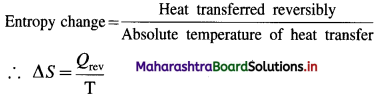(ii) Units of entropy are JK-1 in SI unit and cal K-1 in c.g.s. units. It is also expressed in terms of entropy unit (e.u.). Hence 1 e.u. = 1 JK-1.
(iii) Entropy is a measure of disorder in the system. Higher the disorder, more is entropy of the system.

Question iii.
How will you calculate reaction enthalpy from data on bond enthalpies?
(i) In chemical reactions, bonds are broken in the reactant molecules and bonds are formed in the product molecules.
(ii) Energy is always required to break a chemical bond while energy is always released in the formation of the bond.
(iii) The enthalpy change of a gaseous reactions (ΔfH0) involving substances with covalent bonds can be calculated with the help of bond enthalpies of reactants and products. (In case of solids we need lattice energy or heat of sublimation while in case of liquids we need heat of evaporation.)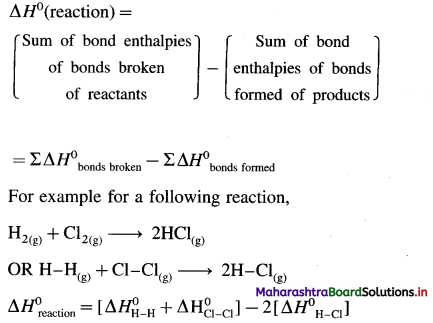If the energy required to break the bonds of reacting molecules is more than the energy released in the bond formation of the products, then the reaction will be endothermic and ∆H0 reaction will be positive. On the other hand if the energy released in the bond formation of the products is more than the energy required to break the bonds of reacting molecules then the reaction will be exothermic and ∆H0 reaction will be negative.

Question iv.
What is the standard enthalpy of combustion ? Give an example.
Standard enthalpy of combustion or standard heat of combustion : it is defined as the enthalpy change when one mole of a substance in the standard state undergoes complete combustion in a sufficient amount of oxygen at constant temperature (298 K) and pressure (1 atmosphere or 1 bar). It is denoted by ∆cH0.
E.g. CH3OH(l) + $$\frac {3}{2}$$ O2(g) = CO2(g) + 2H2O
cH0= -726 kJ mol-1
(∆cH0 is always negative.)
[Note : Calorific value : It is the enthalpy change or amount of heat liberated when one gram of a substance undergoes combustion.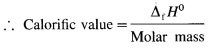Question v.
What is the enthalpy of atomization? Give an example.
Enthalpy of atomisation (∆atomH) : it is the enthalpy change accompanying the dissociation of one mole of gaseous substance into its atoms at constant temperature and pressure.
For example : CH4(g) → C(g) + 4H(g)atomH = 1660 kJ mol-1

Question vi.
Obtain the expression for work done in chemical reaction.
Consider n1 moles of gaseous reactants A of volume V1 change to n2 moles of gaseous products B of volume V2 at temperature T and pressure P.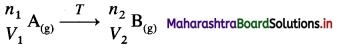In the initial state, PV1 = n1RT
In the final state, PV2 = n2RT
PV2 – PV1 = n2RT – n1RT = (n2 – n1)RT = ∆nRT
where ∆n is the change in number of moles of gaseous products and gaseous reactants.
Due to net changes in gaseous moles, there arises change in volume against constant pressure resulting in mechanical work, -P∆V.
∴ W = -P∆V = -P(V2 – V1) = – ∆nRT
(i) If n1 – n2, ∆n = 0, W = 0. No work is performed.
(ii) If n2 > n1, ∆n > 0, there is a work of expansion by the system and W is negative.
(iii) If n2 < n1, ∆n < 0, there is a work of compression, hence work is done on the system and W is positive.

Question vii.
Derive the expression for PV work.
Consider a certain amount of an ideal gas enclosed in an ideal cylinder fitted with massless, frictionless rigid movable piston at pressure P, occupying volume V1 at temperature T.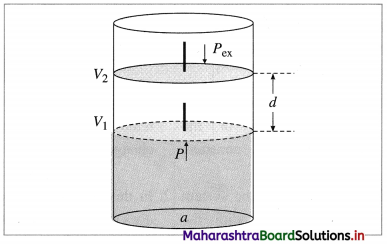Fig. 4.8 : Work of expansion
As the gas expands, it pushes the piston upward through a distance d against external force F, pushing the surroundings.
The work done by the gas is,
W = opposing force × distance = -F × d
-ve sign indicates the lowering of energy of the system during expansion.
If a is the cross section area of the cylinder or piston, then,
W = $$-\frac{F}{a}$$ × d × a
Now the pressure is Pex = $$\frac{F}{a}$$
while volume change is, ΔV = d × a
∴ W = -Pex × ΔV
If during the expansion, the volume changes from V1 and V2 then, ΔV = V2 – V1
∴ W= -Pex(V2 – V1)
During compression, the work W is +ve, since the energy of the system is increased,
W = +Pex(V2 – V1)

Question viii.
What are intensive properties? Explain why density is intensive property.
(A) Intensive property : It is defined as a property of a system whose magnitude is independent of the amount of matter present in the system.
Explanation :

1. Intensive property is characteristic of the system, e.g., refractive index, density, viscosity, temperature, pressure, boiling point, melting point, freezing point of a pure liquid, surface tension, etc.
2. The intensive properties are not additive.

(B) Density is a ratio of two extensive properties namely, mass and volume. Since the ratio of two extensive properties represents an intensive property, density is an intensive property. It does not depend on the amount of a substance.

Question ix.
How much heat is evolved when 12 g of CO reacts with NO2 ? The reaction is :
4 CO(g) + 2 NO2(g) → 4CO2(g) + N2(g), ∆H0 = -1200 kJ4. Answer the following questions.

Question i.
Derive the expression for the maximum work.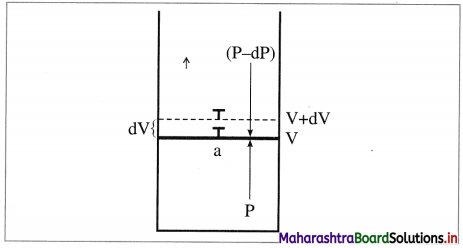Consider n moles of an ideal gas enclosed in an ideal cylinder fitted with a massless and frictionless movable rigid piston. Let V be the volume of the gas at a pressure P and a temperature T.
If in an infinitesimal change pressure changes from P to P – dP and volume increases from V to V + dV. Then the work obtained is, dW = -(P-dP) dV
= -PdV + dPdV
Since dP.dV is negligibly small relative to PdV
dW= -PdV
Let the state of the system change from A(P1, L1) to B (P2, V2) isothermally and reversibly, at temperature T involving number of infinitesimal steps.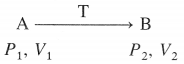Then the total work or maximum work in the process is obtained by integrating above equation.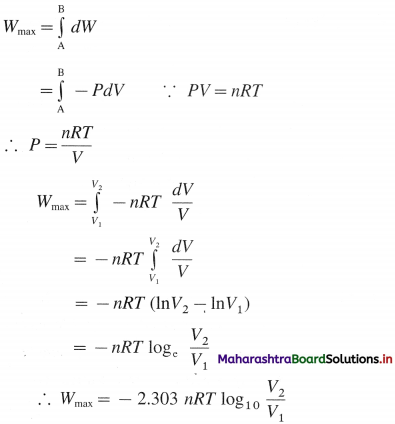At constant temperature,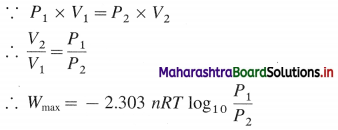where n, P, V and T represent number of moles, pressure, volume and temperature respectively. For the process,
ΔU = 0, ΔH = 0.
The heat absorbed in reversible manner
Qrev, is completely converted into work.
Qrev = -Wmax.
Hence work obtained is maximum.

Question ii.
Obtain the relatioship between ∆H and ∆U for gas phase reactions.
Consider a reaction in which n1 moles of gaseous reactant in initial state change to n2 moles of gaseous product in the final state.
Let H1, U1, P1, V1 and H2, U2, P2, V2 represent enthalpies, internal energies, pressures and volumes in the initial and final states respectively then,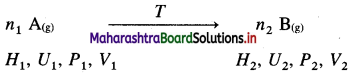The heat of reaction is given by enthalpy change ΔH as,
ΔH = H2 – H1
By definition, H = U + PV
∴ H1 = U1 + P1V1 and H2 = U2+ P2V2
∴ ΔH = (U2 + P2V2) – (U1 + P1V1)
= (U2 – U1) + (P2V2 – P1V1)
Now, ΔU = U2 – U1
Since PV = nRT,
For initial state, P1V1= n1RT
For final state, P2V2 = n2RT
∴ P2V2 – P1V1 = n2RT – n1RT
= (n2 – n1) RT
= ΔnRT
where Δn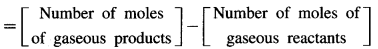∴ ΔH = ΔU + ΔnRT
If QP and QV are the heats involved in the reaction at constant pressure and constant volume respectively, then since QP = ΔH and QV = ΔU.
∴ QP = QV = ΔnRT

Question iii.
State Hess’s law of constant heat summation. Illustrate with an example. State its applications.
Statement of law of constant heat summation : It states that, the heat of a reaction or the enthalpy change in a chemical reaction depends upon initial state of reactants and final state of products and independent of the path by which the reaction is brought about (i.e. in single step or in series of steps).
OR
Heat of reaction is same whether it is carried out in one step or in several steps.
Explanation :
Consider the formation of CO2(g).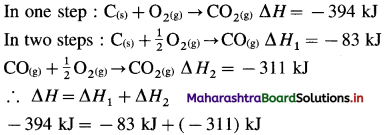Hess’s law treats thermochemical equations mathematically i.e., they can be added, subtracted or multiplied by numerical factors like algebraic equations.

Applications : Hess’s law is used for :

1. To calculate heat of formation, combustion, neutralisation, ionization, etc.
2. To calculate the heat of reactions which may not take place normally or directly.
3. To calculate heats of extremely slow or fast reactions.
4. To calculate enthalpies of reactants and products.Question iv.
Although ∆S for the formation of two moles of water from H2 and O2 is -327 JK-1, it is spontaneous. Explain. (Given ∆H for the reaction is -572 kJ).
Given : ΔS= -327 JK-1; ΔH = -572 kJ
ΔG = ΔH – TΔS, and ΔH << ΔS
∴ ΔG < 0, and hence the formation of H2O(l) is spontaneous.

Question v.
Obtain the relation between ∆G and ∆STotal. Comment on spontaneity of the reaction.
(i) Gibbs free energy, G is defined as,
G = H – TS
where H is the enthalpy, S is the entropy of the system at absolute temperature T.
Since H, T and S are state functions, G is a state function and a thermodynamic function.

(ii) At constant temperature and pressure, change in free energy ΔG for the system is represented as, ∆G = ∆H – T∆S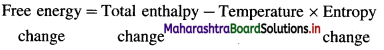This is called Gibbs free energy equation for ∆G. In this ∆S is total entropy change, i.e., ∆STotal.

(iii) The SI units of ∆G are J or kJ (or Jmol-1 or kJmol-1).
The c.g.s. units of ∆G are cal or kcal (or cal mol-1 or kcal mol-1.)

The second law explains the conditions of spontaneity as below :
(i) ∆Stotal > 0 and ∆G < 0, the process is spontaneous.
(ii) ∆Stotal < 0 and ∆G > 0, the process is nonspontaneous.
(iii) ∆Stotal = 0 and ∆G = 0, the process is at equilibrium.

Question vi.
One mole of an ideal gas is compressed from 500 cm3 against a constant external pressure of 1.2 × 105 Pa. The work involved in the process is 36.0 J. Calculate the final volume.
Given : V1 = 500 cm3 = 0.5 dm3;
Pex = 1.2 × 105 Pa = 1.2 bar; W= 36 J;
1 dm3 bar = 100 J; V2 = ?
W = -Pex (V2 – V1)
36 J = – 1.2 (V2 – 0.5) dm3 bar
= -1.2 × 100 (V2 – 0.5) J
∴ V2 – 0.5 = $$\frac{-36}{1.2 \times 100}=-0.3$$
∴ V2 = 0.5 -0.3 = 0.2 dm3 = 200 cm3
Ans. Final volume = 200 cm3.

Question vii.
Calculate the maximum work when 24g of O2 are expanded isothermally and reversibly from the pressure of 1.6 bar to 1 bar at 298 K.
Given : W02 = 24 g, P1 = 1.6 bar, P2 = 1 bar
T = 298 K, Wmax = ?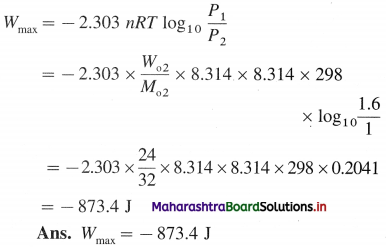Question viii.
Calculate the work done in the decomposition of 132 g of NH4NO3 at 100 °C.
NH4NO3(s) → N2O(g) + 2 H2O(g)
State whether work is done on the system or by the system.
NH44NO3(s) → N2O(g) + 2 H2O(g)
mNH4NO3 = 132 g; MNH4NO3 = 80 g mol-1
T = 273 + 100 = 373 K; Δn = ?
For the reaction,
Δn = Σn2 gaseous products – Σn1 gaseous reactants
= 3 – 0 = 3 mol
Since there is an increase in number of gaseous moles, the work is done by the system.
nNH4NO3 = $$\frac{m_{\mathrm{NH}_{4} \mathrm{NO}_{3}}}{M_{\mathrm{NH}_{4} \mathrm{NO}_{3}}}$$
= $$\frac{132}{80}$$
= 1.65 mol
For 1 mol NH4NO3(s) Δn = 3 mol
∴ For 1.65 mol NH4NO3(s) Δn = 3 × 1.65 = 4.95 mol
W = -ΔnRT = -4.95 × 8.314 × 373
= – 15350 J
= – 15.35 kJ
Ans. Work is done by the system.
Work done = – 15.35 kJQuestion ix.
Calculate standard enthalpy of reaction,
Fe2O3(s) + 3CO(g) → 2 Fe(s) + 3CO2(g),
from the following data.
fH0(Fe2O3) = -824 kJ/mol,
fH0(CO) = -110 kJ/mol,
fH0(CO2) = -393 kJ/mol
Given : ∆fH0Fe2O3 = -824 kJ/mol-1;
fH0(CO) = – 110 kJ mol-1
fH0(CO2) = – 393 kJ/mol-1; ∆fH0 = ?
Required equation,
Fe2O3(s) + 3CO(g) → 2Fe(s) + 3CO2(g)
∆H1 = ? – (I)
Given equations :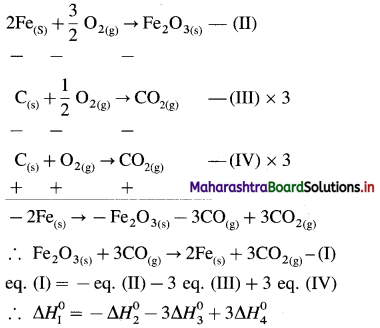= -(-824) -3 (-110) + 3(-393)
= 824 + 330 – 1179
fH0 = -25 kJ
Ans. ∆fH0 = -25 kJ

Question x.
For a certain reaction ∆H0 =219 kJ and ∆S0 = -21 J/K. Determine whether the reaction is spontaneous or nonspontaneous.
Given : ∆H0 = 219 kJ; ∆S0 = -21 J/K, ∆G0 = ?
For standard state, T = 298 K
∆G0 = ∆H0 – T∆S0
= 219 – 298 × (-21) × 10-3
= 219 + 6.258
= 225.3 kJ
Since ∆S < 0 and ∆G0 > 0, the reaction is non-spontaneous.

Question xi.
Determine whether the following reaction is spontaneous under standard state conditions.
2 H2O(l) + O2(g) → 2H2O2(l)
if ∆H0 = 196 kJ, ∆S0 = -126 J/K
Does it have a cross-over temperature?
Given : 2H2O(l) + O2(g) → 2H2O2(l)
∆H0 = +196 kJ
∆S0 = -126 JK-1 =0.126 kj K-1
T= 298 K
∆G0 = ?
Cross over temperature = T = ?
∆G0 = ∆H0 – T∆S0
= 196 – 298 (-0.126)
= 196 + 37.55
= + 233.55 kJ
∵ ∆G0 > 0, the reaction is non-spontaneous.
∆H0 > 0, ∆S0 < 0,
Since at all temperatures, ∆G0 > 0, there is no cross over temperature.
Ans. The reaction is non-spontaneous.
There is no cross-over temperature for the reaction.

Question xii.
Calculate ∆U at 298 K for the reaction,
C2H4(g) + HCl(g) → C2H5Cl(g), ∆H = -72.3 kJ
How much PV work is done?
Given : C2H4(g) + HCl(g) → C2H5Cl(g)
T = 298 K; ∆H = -72.3 kJ; PV = ?;
∆U = ?
∆n = Σn2gaseous products – Σn1gaseous reactants
= 1 – (1 + 1)= -1 mol
For PV work :
W = -∆nRT
= – (- 1) × 8.314 × 298
= 2478 J = 2.478 kJ
∆H = ∆U + ∆nRT
∴ ∆U = ∆H – ∆nRT
= – 72.3 – (-2.478)
= – 69.82 kJ
Ans. PV work = 2.478 kJ
∆U = -69.82 kJ

Question xiii.
Calculate the work done during synthesis of NH3 in which volume changes from 8.0 dm3 to 4.0 dm3 at a constant external pressure of 43 bar. In what direction the work energy flows?
Given : V1 = 8.0 dm3; V2 = 4.0 dm3; Pex = 43 bar
W = ? What direction work energy flows ?
W = -Pex(V2 – V1)
= -43 (4 – 8)
= 172 dm3 bar
= 172 × 100 J
= 17200 J
= 17.2 kJ
In this compression process, the work is done on the system and work energy flows into the system.Question xiv.
Calculate the amount of work done in the
(a) oxidation of 1 mole HCl(g) at 200 °C according to reaction.
4HCl(g) + O2(g) → 2 Cl2(g) + 2 H2O(g)
(b) decomposition of one mole of NO at 300 °C for the reaction
2 NO(g) → N2(g) + O2
Given :
(a) 4HCl(g) + O2(g) → 2Cl2(g) + 2H2O(g)
nHCl = 1 mol; T = 273 + 200 = 473 K, W = ?
For 4 mol HCl ∆n = (2 + 2) – (4 + 1) = – 1 mol
∴ For 1 mol HCl ∆n = –$$\frac {1}{4}$$ = -0.25 mol
W = -∆nRT = – (-0.25) × 8.314 × 473 = 983.11
(b) ∆n = (1 + 1) – 2 = 0 mol
W = -∆nRT = -(0) × 8.314 × 473 = 0
Ans. (a) W = 983.1 J
(b) W = 0.0 J

Question xv.
When 6.0 g of O2 reacts with CIF as per
2CIF(g) + O2(g) → Cl2O(g) + OF2(g)
The enthalpy change is 38.55 kJ. What is standard enthalpy of the reaction ?
Given : The given reaction is for 1 mol O2 or 32 g O2.
∵ For 6.0 g O2
∆ H0 = 38.55 kJ
∴ For 32 g O2
∆ H0 = $$\frac{32 \times 38.55}{6}$$
= 205.6 kJ
Ans. ∆H0 = 205.6 kJ

Question xvi.
Calculate the standard enthalpy of formation of CH3OH(l) from the following data:
i. CH3OH(l) + $$\frac {3}{2}$$ O2(g) → CO2(g) + 2H2O(l), ∆H0 = -726 kJ mol-1
ii. C (Graphite) + O2(g) → CO2(g), ∆cH0 = -393 kJ mol-1
iii. H2(g) + $$\frac {1}{2}$$ O2(g) → H2O(l), ∆fH0 = -286 kJ mol-1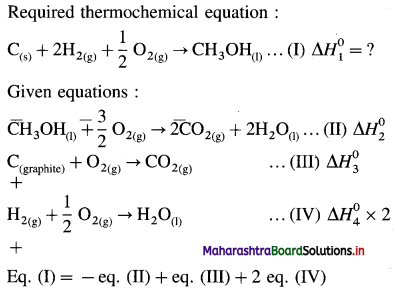∴ ∆H0
= –$$\Delta H_{2}^{0}$$ + $$\Delta H_{3}^{0}$$ + 2∆$$\Delta H_{4}^{0}$$
= – (- 726) + (- 393) + 2(- 286)
= 726 – 393 – 572
= – 239 kJ mol-1
Ans. Standard enthalpy of formation = ∆fH0= -239 kJ mol-1Question xvii.
Calculate ∆H0 for the following reaction at 298 K
H2B4O7(s) + H2O(l) → 4HBO2(aq)
i. 2H3BO3(aq) → B2O3(s) + 3H2O(l), ∆H0 = 14.4 kJ mol-1
ii. H3BO3(aq) → HBO2(aq) + H2O(l), ∆H0 = -0.02 kJ mol-1
iii. H2B4O7(s) → 2B2O3(s) + H2O(l), ∆H0 = 17.3 kJ mol-1
Given equations :
i. 2H3BO3(aq) → B2O3(s) + 3H2O(l), ……….(i)
∆H0 = 14.4 kJ mol-1
ii. H3BO3(aq) → HBO2(aq) + H2O(l) ……….(ii)
∆H0 = -0.02 kJ mol-1
iii. H2B4O7(s) → 2B2O3(s) + H2O(l), ……….(iii)
∆H0 = 17.3 kJ mol-1
Required equation :
(iv) H2B4O7(s) + H2O(l) → 4HBO2(aq) ……. (iv)
$$\Delta H_{4}^{0}=?$$
To obtain eq. (iv) add 4 times equation (ii) and eq.
(iii) and subtract 2 times equation (i).
∴ eq. (iv) = 4 eq. (ii) + eq. (iii) – 2eq. (i)
∴ $$\Delta H_{4}^{0}=4 \Delta H_{2}^{0}+\Delta H_{3}^{0}-2 \Delta H_{1}^{0}$$
= 4(-0.02) + 17.3 – 2(14.4)
= -0.08 + 17.3 – 28.8
= -11.58 kJ
∴ Enthalpy change for the reaction
= ∆rH0 = -11.58 kJ
Ans. ∆rH0 for the given reaction = -11.58 kJ

Question xviii.
Calculate the total heat required (a) to melt 180 g of ice at 0 °C, (b) heat it to 100 °C and then (c) vapourise it at that temperature. Given ∆fusH(ice) = 6.01 kJ mol-1 at 0 °C, ∆vapH(H2O) = 40.7 kJ mol-1 at 100 °C specific heat of water is 4.18 J g-1 K-1.
Given : Mass of ice = m = 180 g
T1 = 273 + 0 °C = 273 K
T2 = 273 + 100 °C = 373 K
fusH(ice) = ∆fusH(H2O)(s) = 6.01 kJ mol-1
vapHH2O(l) = 40.7 kJ mol-1
Specific heat of water = C = 4.18 J g-1 K-1
For converting 180 g ice into vapour, ∆ HTotal = ?
Number of moles of H2O = $$\frac {180}{18}$$ = 10 mol
The total process can be represented as,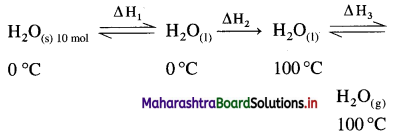(i) ∆H1 = ∆fusH = 10 mol × 6.01 kJ mol-1
= 60.1 kJ
(ii) When the temperature of water is raised from 0 °C to 100 °C (i.e., 273 K to 373 K), then
∆ H2 = m × C × ∆T
= m × C × (T2 – T1)
= 180 g × 4.18 Jg-1K-1 × (373 – 273) × 10-3 kJ = 75.24 kJ
∆ H3 = ∆vapH = 10 mol × 40.7 kJ mol-1 = 407 kJ
Hence total enthalpy change,
∆ HTotal = ∆H1 + ∆H2 + ∆H3
= 60.1 + 75.24 + 407
= 542.34 kJ
Ans. Total heat required = 542.34 kJ

Question xix.
The enthalpy change for the reaction,
C2H4(g) + H2(g) → C2H6(g)
is -620 J when 100 ml of ethylene and 100 mL of H2 react at 1 bar pressure. Calculate the pressure volume type of work and ∆U for the reaction.
Given :
\begin{aligned} &\mathrm{C}_{2} \mathrm{H}_{4(\mathrm{~g})}+\mathrm{H}_{2(\mathrm{~g})} \longrightarrow \mathrm{C}_{2} \mathrm{H}_{6(\mathrm{~g})} \\ &100 \mathrm{~mL} \quad 100 \mathrm{ml} \quad 100 \mathrm{ml} \end{aligned}
∆H = – 620 J; VC2H4 = 100 mL; VH2 = 100 mL
Pex= 1 bar; W=?; ∆U = ?
∆V = 100 – (100 + 100) = -100 mL = -0.1 dm3
W = -Pex(V2 – V1)
= -Pex × ∆V
= -1 × (-0.1)
= 0.1 dm3 bar
= 0.1 × 100 J
= +10 J
∆H = ∆U + P∆V
∴ ∆U = ∆H – P∆V = -620 – (+10) = -610 J
Ans. W = +10 J; ∆U = -610 J

Question xx.
Calculate the work done and comment on whether work is done on or by the system for the decomposition of 2 moles of NH4NO3 at 100 °C
NH4NO3(s) → N2O(g) + 2H2O(g)
Given : NH4NO3(s) → N2O(g) + 2H2O(g)
nNH4NO3 = 2 mol; T = 273 + 100 = 373 K
W = ? Comment on work = ?
∆nreaction = (1 + 2) – 0 = 3 mol
∵ For 1 mol of NH4NO3 ∆nreaction = 3 mol
∴ For 2 mol of NH4NO3 ∆nreaction = 6 mol
Due to 6 moles of gaseous products from 2 mol NH4NO3, there is work of expansion, hence work is done by the system.
W = -∆nRT
= – 6 × 8.314 × 373 = -18606 J
= -18.606 kJ
Ans. Work is done by the system.
W= -18.606 kJ12th Chemistry Digest Chapter 4 Chemical Thermodynamics Intext Questions and Answers

(Textbook page No. 73)

Question 1.
Under what conditions ∆H = ∆U ?
(a) ∆H = ∆U + P∆V
when ∆V = 0, ∆H = ∆U
(b) ∆H = ∆U + ∆nRT
when ∆n = 0, ∆H = ∆U

Try this… (Textbook page No. 71)

Question 1.
25 kJ of work is done on the system and it releases 10 kJ of heat. What is ∆U?
W = 25 kJ; Q= -10 kJ
∆U = Q + W = -10 + 25
∆U = + 15 kJ

Try this… (Textbook page No. 75)

Question 1.
For KCl, ∆LH = 699 kJ/mol-1 and ∆hydH = -681.8 kJ/mol-1. What will be its enthalpy of solution?
Enthalpy of solution :
solnH = ∆LH + ∆hydH
= 699 + (-681.8)
solnH = +17.2 kJ mol-1Try this… (Textbook page No. 76)

Question 1.
Given the thermochemical equation,
C2H2(g) + $$\frac {5}{2}$$ O2(g) → 2CO2(g)+ H2O(l), ∆rH0 = -1300 kJ
Write thermochemical equations when
i. Coefficients of substances are multiplied by 2.
ii. equation is reversed.
(i) 2C2H2(g) + 5O2(g) → 4CO2(g) + 2H2O(l)
rH0 = -2 × 1300 kJ
= – 2600 kJ
(ii) 2CO2(g) + H2O(l) → C2H2(g) + $$\frac {5}{2}$$O2(g)
rH0 = +1300 KJ

Try this… (Textbook page No. 78)

Question 1.
(i) Write thermochemical equation for complete oxidation of one mole of H2(g). Standard enthalpy change of the reaction is -286 kJ.
(ii) Is the value -286 kJ, enthalpy of formation or enthalpy of combustion or both? Explain.
(i) H2(g) + $$\frac {1}{2}$$O2(g) → H2O(l) ∆cH0 = -286 KJ mol-1
(ii) The value -286 kJ is the standard enthalpy of formation of H2O(l) or standard enthalpy of combustion of H2(g).

Question 2.
Write equation for bond enthalpy of Cl-Cl bond in Cl2 molecule ∆rH0 for dissociation of Cl2 molecule is 242.7 kJ.
Equation for bond enthalpy :
Cl2(g) → 2Cl(g)rH0 = 242.7 kJ mol-1
∴ Bond enthalpy of Cl2 = 242.7 kJ mol-1Try this… (Textbook page No. 82)

Question 1.
State whether ∆S is positive, negative or zero for the following reactions.
i. 2H2(g) + O2(g) → 2H2O(l)
ii. CaCO3(s) → CaO(s) + CO2(g)